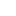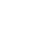# Determination of Calcium and Magnesium ions In hard water

#### Title:

Determination of Calcium and Magnesium ions In hard water by complexometric titration

#### Chemicals required:

• Distilled water
• Hard water sample
• Ethylenediamine Tetra acetic acid
• Ammonium buffer
• Potassium hydroxide
• Calcon indicator
• Eriochrome Black T

#### Apparatus:

• Burette
• Pipette
• Measuring cylinder
• Beaker
• Iron stand

#### Theory:

Determination of calcium and magnesium ion concentration is based on complexometric titration. It is a type of titration that is practically useful for the determination of a mixture of different metal ions in the solution. Many metal ions react with the electron-pair donors to form co-ordinate compounds that are complex in the solution many metal ions react to form coordination complexes. The formation of a particular class of coordination compound called chalets, which is especially well-suited for quantitative methods. A chelate is formed when a metal coordinates with two donor types of a single ligand. A complex is formed when metal ion co-ordinates with ligand like EDTA.

#### EDTA:

EDTA is suitable and can be stored for months and can also be prepared. The need of the fact is that it has 4 protons and always reacts in 1:1 with metals to form complexes.

M+ + EDTA→ [M (EDTA)] 2+

#### Structure of EDTA:

Ethylene di-ammine tetra acetic acid contains four protons asBut its stability constant is very low so to make it completely soluble in the water it is converted into the sodium salt of EDTA which gives the trans product.#### Effect of PH:

Complex formation while using EDTA depends upon the pH of the solution. The pH required for complexes of calcium and magnesium ions is 10.

A reagent that is used in a chemical analysis that reacts with chemical species that may form the interference in the analysis is known as a masking agent. Actually, EDTA reacts with many metals like calcium, magnesium, nickel, zinc, etc at the same time. So the determination of the concentration of one ion becomes impossible without masking agents. It can be possible by using masking agents. Here in the present practical potassium hydroxide is used as a masking agent.

#### Indicator:

Indicators are the organic compounds that are used to indicate the completion of the reaction it shows the color changes at the completion of the reaction. In this experiment, two indicators are used

• Eriochrome black T
• Calcon

#### Eriochrome black T:

Eriochrome Black T is an azo dye. It is used in the complexometric titration. Its chemical formula is C20H12N3NaO7S. Its molecular mass is 460 1.38 grams per mole it is dark brown in color.#### Calcon:

Calcon is also so can as a dye. Its molecular formula is C21H14N2O7S . It reacts with EDTA at the pH of 12.25.#### Endpoint:

There are two indicators that have different endpoint

• EBT Wine red to blue
• Calcon Pink to blue

#### Procedure:

1st step

• Glass where was washed and cleaned carefully
• The burette was filled with 0.01 m EDTA solution
• 10 ml of sample was taken in titration flask with the help of a pipette
• Distilled water was added to it
• 1-2 ml of ammonical buffer solution was added to the sample
• A few crystals of EBT were added to the conical flask
• It was titrated against the EDTA solution taken in burette to find the exact value of standard solution used, was determined
• The procedure was repeated three times to get the 3 readings

Second step

• The conical flask was first washed and dried carefully with distilled water
• 10 ml of sample was taken in this conical flask and diluted with distilled water
• About 1-2 ml of potassium hydroxide was added which acts as a masking agent. In this experiment, Mg+ ions are masked by potassium hydroxide
• A few crystals of indicator Calcon indicator were added
• Now it was titrated against the standard solution taken in the burette
• Similarly, three readings were taken to get the mean value

#### Observations and calculations:

Molarity of EDTA = 0.01 m

The volume of sample solution taken = 10 ml

 Sr. No Initial reading ml Final reading ml Volume used ml 1 0.0 19.0 19.0 2 19.0 38.2 19.2 3 38.2 57.3 19.1

Mean value = 19+19.2+19.1/3

= 19.1 ml

 Sr. No Initial reading ml Final reading ml Volume used Ml 1 0.0 9.3 9.3 2 9.3 18.2 8.9 3 18.2 27.4 9.2

Mean volume = 9+38.9+9.2/3

= 9.3 ml

Volume of EDTA used for EBT (Ca2+, Mg2+) = 19.1ml

Volume of EDTA used for Calcon Ca2+ =9.13 ml

Volume of EDTA used for Mg2+ =19.1 − 9.13

=9.91 ml

The molarity of Ca2+

EDTA = Sample

M1V1 /N1= M2V2 /N2

0.01× 9.13/1 = M2 × 10/1

M2 = 0.00913 m

The Strength of Ca2+

Strength / L = molarity × molecular mass

= 0.000913 × 40

= 0.365 g/L

The molarity of Mg2+

EDTA = Sample

M1V1 /N1= M2V2 /N2

0.01 × 9.91 / 1 = M2 × 10 /1

M2 = 0.0093 m

The strength of Mg2+

Strength / L = molarity × molecular mass

= 0.0093 × 24

= 0.239 g/L

#### Result:

• The strength of calcium ions in the sample was 0.365 gram per liter
• The n strength of magnesium ions in the sample was 0.239 grams per liter

#### Precautions:

• The glassware should wash with tap water first and then rinse with distilled water because tap water also contains the ions of metals like calcium magnesium nickel etc
• Burette should be set dropwise in order to get the exact readings

Determination of Calcium and Magnesium ions In hard water by complexometric titration###### Don’t miss these Chemistry topics!
Sharing Is Caring:

### 13 thoughts on “Determination of Calcium and Magnesium ions In hard water”

1.Very Nice Superbbbbbbbbb Work

2.Wow…. it’s an amazing site

•Thank you so much

3.Good work

4.MASHA ALLAH….
Very interesting and informative….
💫💫💫

•thank you

5.Nice work

6.So nice work

7.Simplified explanations
Keep this good work up
May Allah bless u

•thank you so much

8.Нurrɑh, that’s ԝhat І was exploring for, what a material!
existіng here at this webpage, thanks aԁmіn of this web page.

•9.